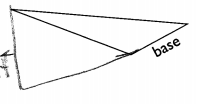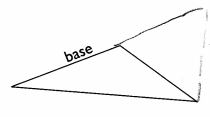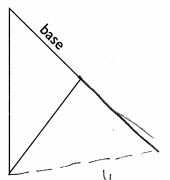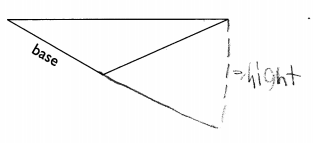# Math in Focus Grade 5 Chapter 6 Practice 2 Answer Key Base and Height of a Triangle

Practice the problems of Math in Focus Grade 5 Workbook Answer Key Chapter 6 Practice 2 Base and Height of a Triangle to score better marks in the exam.

## Math in Focus Grade 5 Chapter 6 Practice 2 Answer Key Base and Height of a Triangle

Complete to give both the base and the height in each triangle.

Example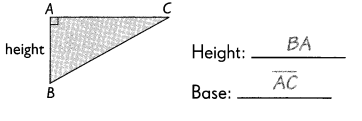Question 1.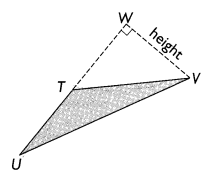Height: _____
Base: ______
From the above figure Base of the triangle is UT. Height of the triangle is WV.

Question 2.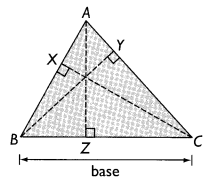Base: ______
Height: _____
From the above figure Base of the triangle is BC. Height of the triangle is ZA.

Question 3.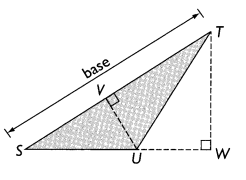Base: ____
Height: ____
From the above figure Base of the triangle is ST. Height of the triangle is VU.

Question 4.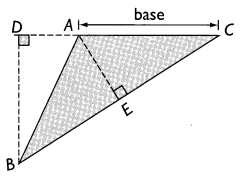Base: ____
Height: ____
From the above figure Base of the triangle is AC. Height of the triangle is DB.

For each triangle, the base is given.
Use a drawing triangle to draw the height. Label the height.

Example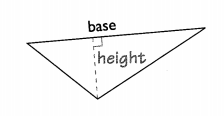Question 5.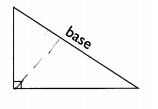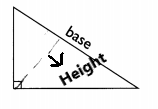Explanation:

Question 6.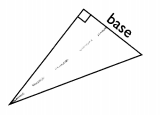Question 7.